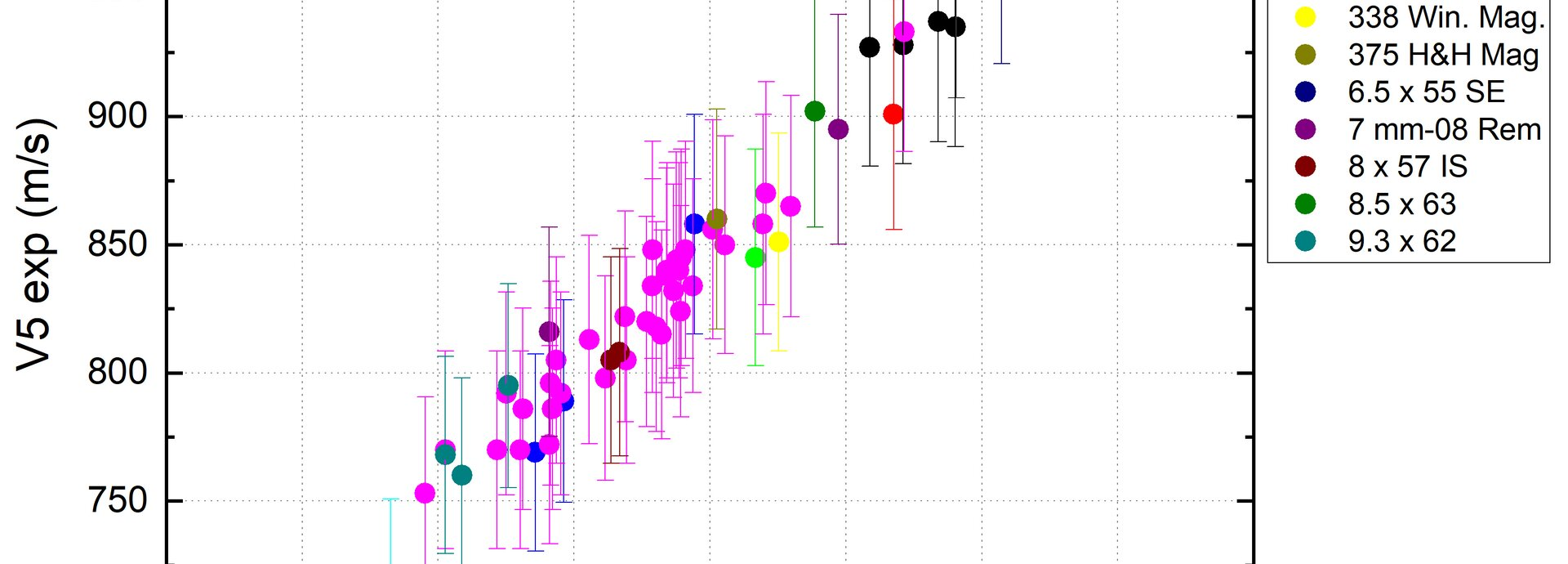jumpToMain# 05.05.2021 | Hints from the experts

Mathematical modeling with RS52

Dear users,

We are currently exploring multivariate statistics in order to identify interrelationships. As a part of our project work, we are focusing on customer load data with RS52. This propellent is extremely popular, with well over 100 loads already in the second database. That means we have enough datasets to calibrate our model. We have selected the following variables as inputs for the model:

• Bullet weight
• Charge mass
• Barrel length
• Cartridge length L6 (C.O.L.)
• Barrel cross-section according to CIP values 

As our output parameter, we have defined the bullet velocity 5 m from the muzzle (V5). Given our understanding of the physics, the following effects were assumed:

• The greater the bullet weight, the lower the velocity (-)
• The greater the charge mass, the higher the velocity (+)
• The longer the barrel, the higher the velocity (+)
• The longer the cartridge, the greater the volume in the case, the lower the velocity (-)
• The greater the barrel cross-section, the lower the velocity (-)

A multiple linear regression with 57 datasets  and a correlation coefficient of 0.97  resulted in the following equation for propellent RS52:

V5 = A + a * mc + b * mbu + c * lb + d * lp + e * Q

Variables and values for the coefficients
Variable Meaning Unit Calibration range Coefficient Coefficient value
mc Powder mass grains 26 – 72 a 13.53
mbu Bullet weight grains 63 – 250 b -1.48
lb Barrel length mm 57 – 91 c 0.25
lp Cartridge length mm 420 - 813 d -6.37
Q Area of cross-section mm2 25 – 70 e -4.91
A y-axis section m/s - A 1011